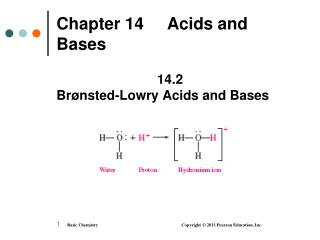DownloadDownload PresentationChapter 14 Acids and Bases

# Chapter 14 Acids and Bases

Télécharger la présentation## Chapter 14 Acids and Bases

- - - - - - - - - - - - - - - - - - - - - - - - - - - E N D - - - - - - - - - - - - - - - - - - - - - - - - - - -
##### Presentation Transcript

1. Chapter 14 Acids and Bases 14.2 Brønsted-Lowry Acids and Bases

2. Hydronium Ion In water, a dissociated proton (H+) bonds to a water molecule to form a hydronium ion, H3O+

3. BrØnsted-Lowry Acids and Bases According to the Brønsted-Lowry theory, • an acid donates a proton (H+) • a base accepts a proton (H+)

4. NH3, A Brønsted-Lowry Base In the reaction of ammonia (NH3) and water, • NH3 acts as a base that accepts H+ • H2O acts as an acid that donates H+

5. Conjugate Acid-Base Pairs In an acid-base reaction, there are two conjugate acid-base pairs: • an acid donates H+ toform its conjugate base • a base accepts H+ to form its conjugate acid • each is related by the loss and gain of H+ • conjugate acid-base pair 1 HA + B A− + BH+ conjugate acid-base pair 2

6. Conjugate Acid-Base Pairs The conjugate acid-base pairs of HF/F− and H3O+/H2O are related by a loss or gain of H+.

7. Conjugate Acid-Base Pairs In this proton-transfer reaction, • the conjugate acid-base pairs are NH4+/NH3 and H2O/OH

8. Comparing Arrhenius and Bronsted Lowry Theories of Acids and Bases • http://www.youtube.com/watch?v=ph5lqm5fi8s

9. Learning Check A. Write the conjugate base of the following: 1. HBr 2. H2S 3. H2CO3 B. Write the conjugate acid of the following: 1. NO2 2. NH3 3. OH

10. Solution A. Remove a H+ to write the conjugate base: 1. HBr Br- 2. H2S HS 3. H2CO3 HCO3 B. Add a H+to write the conjugate acid: 1. NO2 HNO2 2. NH3 NH4+ 3. OH H2O

11. Some Conjugate Acid-Base Pairs

12. Learning Check Identify the sets of acid-base conjugate pairs. 1. HNO2, NO2 2. H2CO3, CO32 3. HCl, ClO4 4. HS, H2S 5. NH3, NH4+

13. Solution An acid-base conjugate pair is related by the loss and gain of H+. 1. HNO2, NO2 4. HS, H2S 5. NH3, NH4+

14. Learning Check A. The conjugate base of HCO3 is 1) CO32 2) HCO3 3) H2CO3 B. The conjugate acid of HCO3 is 1) CO32 2) HCO3 3) H2CO3 C. The conjugate base of H2O is 1) OH 2) H2O 3) H3O+ D. The conjugate acid of H2O is 1) OH 2) H2O 3) H3O+

15. Solution A. The conjugate base of HCO3 is 1) CO32 B. The conjugate acid of HCO3 is 3) H2CO3 C. The conjugate base of H2O is 1) OH D. The conjugate acid of H2O is 3) H3O+